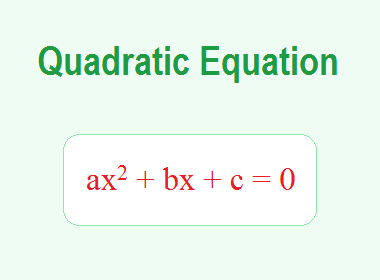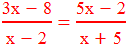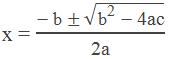The definition of quadratic equation is: “A second degree equation in one variable is known as a Quadratic Equation.”

**********************

10 Math Problems officially announces the release of Quick Math Solver, an android APP on the Google Play Store for students around the world.**********************

x2 – 25 = 0, 4x2 – 81 = 0, x2 + 3x + 2 = 0, 2x2 – 7x – 15 = 0 are some of the examples of quadratic equations.

### Standard Form of Quadratic Equation

A quadratic equation of the form ax2 + bx + c = 0 where a, b and c are constant and a ≠ 0, is called Standard Form of Quadratic Equation. The standard form of quadratic equation is also known as General Form of Quadratic Equation.

3x2 – 5x + 2 = 0 is an example of quadratic equation in standard form.

On the basis of their forms, quadratic equations are of two types:

A quadratic equation of the form ax2 + c = 0 where the middle term bx is missing, is known as a Pure Quadratic Equation. For example: x2 – 9 = 0, 2x2 – 5 = 0 etc. are pure quadratic equations.

A quadratic equation in its standard form is known as an Adfected Quadratic equation. For examples: x2 + 9x + 14 = 0, 2x2 + 5x – 3 = 0 etc. are the adfected quadratic equations.

Solving a quadratic equation means finding the values of the variable that satisfies the quadratic equation.

The values of the variable that satisfies the quadratic equation are called the solution of the quadratic equation. A quadratic equation has degree 2, therefore there are 2 solutions of a quadratic equation.

Two solutions of a quadratic equation are called the roots of the quadratic equation. The roots of a quadratic equation ax2 + bx + c = 0 may be distinct and real, equal and real, or both imaginary (complex number) according to the conditions given below:

1.     When b2 > 4ac, the roots are distinct and real number.

2.     When b2 = 4ac, the roots are equal and real number.

3.     When b2 < 4ac, roots are imaginary or there is no real solution.

### Ways to Solve Quadratic Equations

We can solve a quadratic equation by following three ways:

1.     By factoring quadratic equation method

2.     By completing square method

3.     By using quadratic equation formula

## Solving Quadratic Equations by Factoring

This is the easiest method to solve a quadratic equation. In this method, we factorize the quadratic expression into the product of two linear binomial factors. Then, by applying the zero product property, we can find the solutions of the quadratic equation.

#### What is zero product property?

If the product of any two numbers is 0, then either one or both of the numbers must be 0. This is called Zero Product Property. i.e. if p×q = 0, then either p = 0 or q = 0.

Therefore, after factoring the quadratic expression, we equate each linear binomial factor with 0 and solve it to get the value of variables.

#### Here are the steps for solving quadratic equations by factoring:

Step 1: Remove the brackets of fractions if there is any in the equation.

Step 2: Simplify and reduce the equation in the form of ax2 + bx + c = 0.

Step 3: Factorize the left-hand side into two factors.

Step 4: Use the above property of numbers and write each factor equal to 0.

Step 5: Solve both factors and get two values of the variable.

Here are the examples of solving quadratic equations by factoring:

### Worked Out Examples

Example 1:  Calculate roots of quadratic equation x2 – 9 = 0.

Solution: Here,

x2 – 9 = 0

or,     x2 – 32 = 0

or,     (x + 3)(x – 3) = 0

Either, x + 3 = 0  or, x = - 3

Or, x – 3 = 0  or, x = 3

Example 2:  Calculate roots of quadratic equation 2x2 = 8.

Solution: Here,

2x2 = 8

or,     x2 = 8/2

or,     x2 = 4

or,     x2 – 4 = 0

or,     x2 – 22 = 0

or,     (x + 2)(x – 2) = 0

Either, x + 2 = 0  or, x = - 2

Or, x – 2 = 0  or, x = 2

Example 3:  Calculate roots of quadratic equation x2 – x – 6 = 0.

Solution: Here,

x2 – x – 6 = 0

or,     x2 – (3 – 2)x – 6 = 0

or,     x2 – 3x + 2x – 6 = 0

or,     x(x – 3) + 2(x – 3) = 0

or,     (x – 3)(x + 2) = 0

Either, x 3 = 0  or, x = 3

Or, x + 2 = 0  or, x = –2

Example 4: Find the roots of the following quadratic equation by factorisatione: x2 – 3ax + 2bx – 6ab = 0.

Solution: Here,

x2 – 3ax + 2bx – 6ab = 0

or,     x(x – 3a) + 2b(x – 3a) = 0

or,     (x – 3a)(x + 2b) = 0

Either, x – 3a = 0 or, x = 3a

Or, x + 2b = 0 or, x = -2b

Example 5: Find the roots of the following quadratic equation by factorisation:Solution: Here,or,     (3x – 8)(x + 5) = (5x – 2)(x – 2)

or,     3x2 + 15x – 8x – 40 = 5x2 – 10x – 2x + 4

or,     3x2 + 7x – 40 – 5x2 + 12x – 4 = 0

or,     -2x2 + 19x – 44 = 0

or,     -(2x2 – 19x + 44) = 0

or,     2x2 – 11x – 8x + 44 = 0/-1

or,     x(2x – 11) – 4(2x – 11) = 0

or,     (2x – 11)(x – 4) = 0

Either, x 4 = 0  or, x = 4

Or, 2x – 11 = 0  or, 2x = 11 or x = 11/2

Example 6: Find the roots of the following quadratic equation by factorisation:Solution: Here,or,     5(x2 – 1) = 24x

or,     5x2 – 5 = 24x

or,     5x2 – 24x – 5 = 0

or,     5x2 – (25 – 1)x – 5 = 0

or,     5x2 – 25x + x – 5 = 0

or,     5x(x – 5) + 1(x – 5) = 0

or,     (x – 5)(5x + 1) = 0

Either, x 5 = 0  or, x = 5

Or, 5x + 1 = 0  or, 5x = –1 or x = –1/5

The meaning of quadratic is "power 2". It came from the Latin word "quadratum" meaning "square".

#### What is the meaning of quadratic equation?

The meaning of a quadratic equation is an equation in which there is a quadratic or square of the variable term in the equation.

#### What is the standard form of quadratic equation?

The standard form of quadratic equation is ax2 + bx + c = 0.

#### What is the general form of quadratic equation?

The general form of the quadratic equation is ax2 + bx + c = 0.

#### What are the roots of a quadratic equation?

The roots of a quadratic equation are the solution of the quadratic equation i.e the values of the variable that satisfies the quadratic equation.

#### What is the degree of quadratic equation?

The degree of quadratic equation is 2.

#### What are quadratic equations used for?

The quadratic equations are used to solve the problems related to the area of rectangular field, surrounding-path, cross-path in which the length or breadth are given in terms of variable term, and some conditional problems involving the square of a variable or the product of two variables along with the sum and/or difference.

#### What is the formula of quadratic equation?

The formula of quadratic equation is:If you have any questions or problems regarding the Quadratic Equations and Factorization Method, you can ask here, in the comment section below.